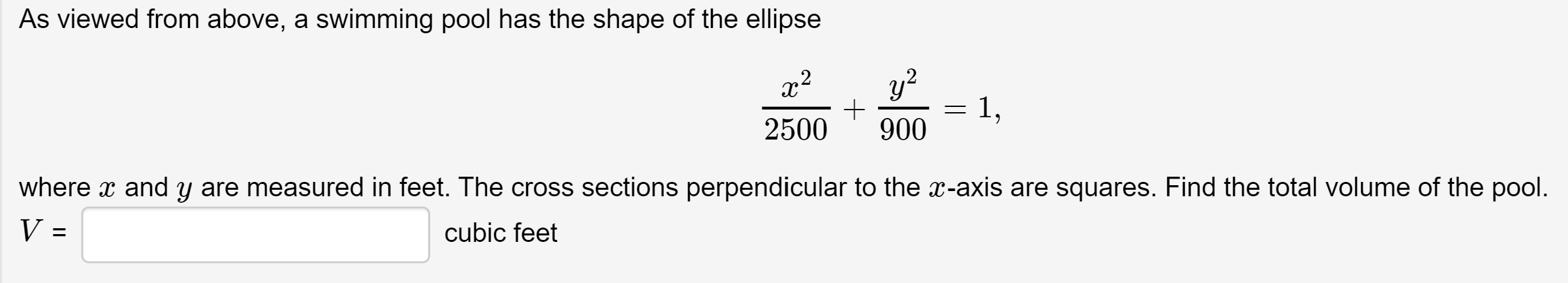# As viewed from above, a swimming pool has the shape of the ellipsey?1,9002500where x and y are measured in feet. The cross sections perpendicular to the x-axis are squares. Find the total volume of the pool.V =cubic feet

Question
4 viewshelp_outlineImage TranscriptioncloseAs viewed from above, a swimming pool has the shape of the ellipse y? 1, 900 2500 where x and y are measured in feet. The cross sections perpendicular to the x-axis are squares. Find the total volume of the pool. V = cubic feet fullscreen
check_circle

Step 1

Suppose that the x and y axes are places so that the origin is at the center of the pool. Observe that each vertical strip perpendicular to the x-axis is in the shape of a square.

Because we have the origin of our coordinate system in the center of the pool, the y-coordinate for each value of x equals half o...

### Want to see the full answer?

See Solution

#### Want to see this answer and more?

Solutions are written by subject experts who are available 24/7. Questions are typically answered within 1 hour.*

See Solution
*Response times may vary by subject and question.
Tagged in

### Calculus